Home Practice
For learners and parents For teachers and schools
Textbooks
Full catalogue
Pricing SupportLog in

We think you are located in United States. Is this correct?

# 13.6 Standard electrode potentials

## 13.6 Standard electrode potentials (ESCRF)

### Standard conditions (ESCRG)

Standard electrode potentials are a measurement of equilibrium potentials. The position of this equilibrium can change if you change some of the conditions (e.g. concentration, temperature). It is therefore important that standard conditions be used:

• pressure = $$\text{101,3}$$ $$\text{kPa}$$ ($$\text{1}$$ $$\text{atm}$$)

• temperature = $$\text{298}$$ $$\text{K}$$ ($$\text{25}$$ $$\text{℃}$$)

• concentration = $$\text{1}$$ $$\text{mol·dm^{-3}}$$

### The standard hydrogen electrode (ESCRH)

It is the potential difference (recorded as a voltage) between the two electrodes that causes electrons to flow from the $$\color{blue}{\textbf{anode}}$$ to the $$\color{red}{\textbf{cathode}}$$ through the external circuit of a galvanic cell (remember, conventional current goes in the opposite direction).

It is possible to measure the potential of an electrode and electrolyte. It is not a simple process however, and the value obtained will depend on the concentration of the electrolyte solution, the temperature and the pressure.

A way to remove these inconsistencies is to compare all electrode potentials to a standard reference electrode. These comparisons are all done with the same concentrations, temperature and pressure. This means that these values can be used to calculate the potential difference between two electrodes. It also means that electrode potentials can be compared without the need to construct the specific cell being studied.

This reference electrode can be used to calculate the relative electrode potential for a substance. The reference electrode that is used is the standard hydrogen electrode (Figure 13.8).

Standard hydrogen electrode

The standard hydrogen electrode is a redox electrode which forms the basis of the scale of oxidation-reduction potentials.

The standard hydrogen electrode consists of a platinum electrode in a solution containing $$\text{H}^{+}$$ ions. The solution (e.g. $$\text{H}_{2}\text{SO}_{4}$$) has a concentration of $$\text{1}$$ $$\text{mol·dm^{-3}}$$. As the hydrogen gas bubbles over the platinum electrode, the reaction is as follows:

$$2\text{H}^{+}(\text{aq}) + 2\text{e}^{-}$$ $$\rightleftharpoons$$ $$\text{H}_{2}(\text{g})$$

The standard hydrogen electrode used now is actually the potential of a platinum electrode in a theoretical acidic solution.

The electrode potential of the hydrogen electrode at $$\text{25}$$ $$\text{℃}$$ is estimated to be $$\text{4,4}$$ $$\text{V}$$. However, in order to use this as a reference electrode this value is set to zero at all temperatures so that it can be compared with other electrodes.

### Standard electrode potentials (ESCRJ)

In order to use the hydrogen electrode, it needs to be attached to the electrode system that you are investigating. For example, if you are trying to determine the electrode potential of copper, you will need to connect the copper half-cell to the hydrogen electrode; if you are trying to determine the electrode potential of zinc, you will need to connect the zinc half-cell to the hydrogen electrode and so on. Let's look at the examples of zinc and copper in more detail.

Zinc

Zinc has a greater tendency than hydrogen to form ions (to be oxidised), so if the standard hydrogen electrode is connected to the zinc half-cell, the $$\color{blue}{\textbf{zinc}}$$ will be relatively $$\color{blue}{\textbf{more negative}}$$ because the electrons that are released when zinc is oxidised will accumulate on the metal.

$$\text{Zn}^{2+}(\text{aq}) + 2\text{e}^{-}$$ $$\to$$ $$\text{Zn}(\text{s})$$

$$2\text{H}^{+}(\text{aq}) + 2\text{e}^{-}$$ $$\to$$ $$\text{H}_{2}(\text{g})$$

The solid zinc is more likely to form zinc ions than the hydrogen gas is to form ions. A simplified representation of the cell is shown in Figure 13.9.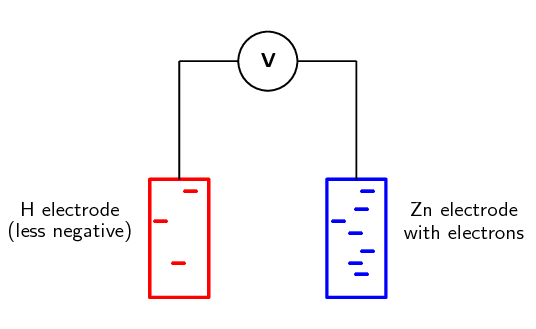Figure 13.9: When zinc is connected to the standard hydrogen electrode, relatively few electrons build up on the platinum (hydrogen) electrode. There are lots of electrons on the zinc electrode.

When determining standard electrode reduction potentials the standard hydrogen electrode is considered to be on the left ($$\text{Pt}(\text{s})|\text{H}_{2}(\text{g}),\text{H}^{+}(\text{aq})||$$). So a negative value means that the other element or compound has a greater tendency to oxidise, and a positive value means that the other element or compound has a greater tendency to be reduced.

The voltmeter measures the potential difference between the charge on these electrodes. In this case, the voltmeter would read $$-\text{0,76}$$ $$\text{V}$$ as the $$\text{Zn}$$ electrode has a relatively higher number of electrons.

Copper

Copper has a lower tendency than hydrogen to form ions, so if the standard hydrogen electrode is connected to the copper half-cell, the $$\color{red}{\textbf{copper}}$$ will be relatively $$\color{red}{\textbf{less negative}}$$.

$$\text{Cu}^{2+}(\text{aq}) + 2\text{e}^{-}$$ $$\to$$ $$\text{Cu}(\text{s})$$

$$2\text{H}^{+}(\text{aq}) + 2\text{e}^{-}$$ $$\to$$ $$\text{H}_{2}(\text{g})$$

The copper ions are more likely to form solid copper than the hydrogen ions are to form hydrogen gas. A simplified representation of the cell is shown in Figure 13.10.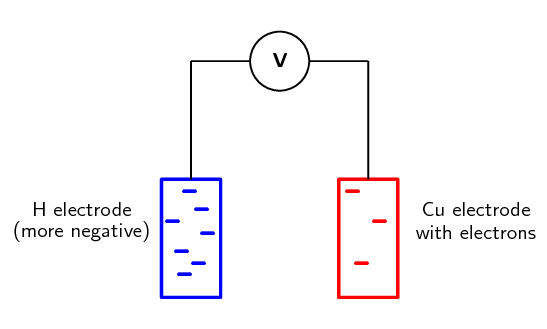Figure 13.10: When copper is connected to the standard hydrogen electrode, relatively few electrons build up on the copper electrode. There are lots of electrons on the hydrogen electrode.

The voltmeter measures the potential difference between the charge on these electrodes. In this case, the voltmeter would read $$\text{+0,34}$$ $$\text{V}$$ as the $$\text{Cu}$$ electrode has a relatively lower number of electrons.

The voltages recorded when zinc and copper were connected to a standard hydrogen electrode are in fact the standard electrode potentials for these two metals. It is important to remember that these are not absolute values, but are potentials that have been measured relative to the potential of hydrogen if the standard hydrogen electrode is taken to be zero.

By convention, we always write the reduction half-reaction when giving the standard electrode potential.

In the examples we used earlier, zinc's electrode reduction potential is $$-\text{0,76}$$ and copper's is $$\text{+0,34}$$. So, if an element or compound has a negative standard electrode reduction potential, it means it forms ions easily. The more negative the value, the easier it is for that element or compound to form ions (be oxidised, and be a reducing agent). If an element or compound has a positive standard electrode potential, it means it does not form ions as easily.

Luckily for us, we do not have to determine the standard electrode potential for every metal. This has been done already and the results are recorded in a table of standard electrode potentials. Table 13.2 is presented as the standard electrode reduction potentials.

 Half-Reaction E° V $$\text{Li}^{+} + \text{e}^{-}$$ $$\rightleftharpoons$$ $$\text{Li}$$ $$-\text{3,05}$$ $$\text{K}^{+} + \text{e}^{-}$$ $$\rightleftharpoons$$ $$\text{K}$$ $$-\text{2,93}$$ $$\text{Ba}^{2+} + 2\text{e}^{-}$$ $$\rightleftharpoons$$ $$\text{Ba}$$ $$-\text{2,90}$$ $$\text{Ca}^{2+} + 2\text{e}^{-}$$ $$\rightleftharpoons$$ $$\text{Ca}$$ $$-\text{2,87}$$ $$\text{Na}^{+} + \text{e}^{-}$$ $$\rightleftharpoons$$ $$\text{Na}$$ $$-\text{2,71}$$ $$\text{Mg}^{2+} + 2\text{e}^{-}$$ $$\rightleftharpoons$$ $$\text{Mg}$$ $$-\text{2,36}$$ $$\text{Al}^{3+} + 3\text{e}^{-}$$ $$\rightleftharpoons$$ $$\text{Al}$$ $$-\text{1,66}$$ $$\text{Mn}^{2+} + 2\text{e}^{-}$$ $$\rightleftharpoons$$ $$\text{Mn}$$ $$-\text{1,18}$$ $$2\text{H}_{2}\text{O} + 2\text{e}^{-}$$ $$\rightleftharpoons$$ $$\text{H}_{2}(\text{g}) + 2\text{OH}^{-}$$ $$-\text{0,83}$$ $$\text{Zn}^{2+} + 2\text{e}^{-}$$ $$\rightleftharpoons$$ $$\text{Zn}$$ $$-\text{0,76}$$ $$\text{Cr}^{3+} + 3\text{e}^{-}$$ $$\rightleftharpoons$$ $$\text{Cr}$$ $$-\text{0,74}$$ $$\text{Fe}^{2+} + 2\text{e}^{-}$$ $$\rightleftharpoons$$ $$\text{Fe}$$ $$-\text{0,44}$$ $$\text{Cr}^{3+} + \text{e}^{-}$$ $$\rightleftharpoons$$ $$\text{Cr}^{2+}$$ $$-\text{0,41}$$ $$\text{Cd}^{2+} + 2\text{e}^{-}$$ $$\rightleftharpoons$$ $$\text{Cd}$$ $$-\text{0,40}$$ $$\text{Co}^{2+} + 2\text{e}^{-}$$ $$\rightleftharpoons$$ $$\text{Co}$$ $$-\text{0,28}$$ $$\text{Ni}^{2+} + 2\text{e}^{-}$$ $$\rightleftharpoons$$ $$\text{Ni}$$ $$-\text{0,27}$$ $$\text{Sn}^{2+} + 2\text{e}^{-}$$ $$\rightleftharpoons$$ $$\text{Sn}$$ $$-\text{0,14}$$ $$\text{Pb}^{2+} + 2\text{e}^{-}$$ $$\rightleftharpoons$$ $$\text{Pb}$$ $$-\text{0,13}$$ $$\text{Fe}^{3+} + 3\text{e}^{-}$$ $$\rightleftharpoons$$ $$\text{Fe}$$ $$-\text{0,06}$$ $$2\text{H}^{+} + 2\text{e}^{-}$$ $$\rightleftharpoons$$ $$\text{H}_{2}(\text{g})$$ 0,00 $$\text{S} + 2\text{H}^{+} + 2\text{e}^{-}$$ $$\rightleftharpoons$$ $$\text{H}_{2}\text{S}(\text{g})$$ $$\text{+0,14}$$ $$\text{Sn}^{4+} + 2\text{e}^{-}$$ $$\rightleftharpoons$$ $$\text{Sn}^{2+}$$ $$\text{+0,15}$$ $$\text{Cu}^{2+} + \text{e}^{-}$$ $$\rightleftharpoons$$ $$\text{Cu}^{+}$$ $$\text{+0,16}$$ $$\text{SO}_{4}^{2-} + 4\text{H}^{+} + 2\text{e}^{-}$$ $$\rightleftharpoons$$ $$\text{SO}_{2}(\text{g}) + 2\text{H}_{2}\text{O}$$ $$\text{+0,17}$$ $$\text{Cu}^{2+} + 2\text{e}^{-}$$ $$\rightleftharpoons$$ $$\text{Cu}$$ $$\text{+0,34}$$ $$2\text{H}_{2}\text{O} + \text{O}_{2} + 4\text{e}^{-}$$ $$\rightleftharpoons$$ $$4\text{OH}^{-}$$ $$\text{+0,40}$$ $$\text{Cu}^{+} + \text{e}^{-}$$ $$\rightleftharpoons$$ $$\text{Cu}$$ $$\text{+0,52}$$ $$\text{I}_{2} + 2\text{e}^{-}$$ $$\rightleftharpoons$$ $$2\text{I}^{-}$$ $$\text{+0,54}$$ $$\text{O}_{2}(\text{g}) + 2\text{H}^{+} + 2\text{e}^{-}$$ $$\rightleftharpoons$$ $$\text{H}_{2}\text{O}_{2}$$ $$\text{+0,68}$$ $$\text{Fe}^{3+} + \text{e}^{-}$$ $$\rightleftharpoons$$ $$\text{Fe}^{2+}$$ $$\text{+0,77}$$ $$\text{NO}_{3}^{-} + 2\text{H}^{+} + \text{e}^{-}$$ $$\rightleftharpoons$$ $$\text{NO}_{2}(\text{g}) + \text{H}_{2}\text{O}$$ $$\text{+0,80}$$ $$\text{Ag}^{+} + \text{e}^{-}$$ $$\rightleftharpoons$$ $$\text{Ag}$$ $$\text{+0,80}$$ $$\text{Hg}^{2+} + 2\text{e}^{-}$$ $$\rightleftharpoons$$ $$\text{Hg}(\text{ℓ})$$ $$\text{+0,85}$$ $$\text{NO}_{3}^{-} + 4\text{H}^{+} + 3\text{e}^{-}$$ $$\rightleftharpoons$$ $$\text{NO}(\text{g}) + 2\text{H}_{2}\text{O}$$ $$\text{+0,96}$$ $$\text{Br}_{2} + 2\text{e}^{-}$$ $$\rightleftharpoons$$ $$2\text{Br}^{-}$$ $$\text{+1,07}$$ $$\text{O}_{2}(\text{g}) + 4\text{H}^{+} + 4\text{e}^{-}$$ $$\rightleftharpoons$$ $$2\text{H}_{2}\text{O}$$ $$\text{+1,23}$$ $$\text{MnO}_{2} + 4\text{H}^{+} + 2\text{e}^{-}$$ $$\rightleftharpoons$$ $$\text{Mn}^{2+} + 2\text{H}_{2}\text{O}$$ $$\text{+1,23}$$ $$\text{Cr}_{2}\text{O}_{7}^{2-} + 14\text{H}^{+} + 6\text{e}^{-}$$ $$\rightleftharpoons$$ $$2\text{Cr}^{3+} + 7\text{H}_{2}\text{O}$$ $$\text{+1,33}$$ $$\text{Cl}_{2} + 2\text{e}^{-}$$ $$\rightleftharpoons$$ $$2\text{Cl}^{-}$$ $$\text{+1,36}$$ $$\text{MnO}_{4}^{-} + 8\text{H}^{+} + 5\text{e}^{-}$$ $$\rightleftharpoons$$ $$\text{Mn}^{2+} + 4\text{H}_{2}\text{O}$$ $$\text{+1,51}$$ $$\text{Au}^{3+} + 3\text{e}^{-}$$ $$\rightleftharpoons$$ $$\text{Au}$$ $$\text{+1,52}$$ $$\text{Co}^{3+} + \text{e}^{-}$$ $$\rightleftharpoons$$ $$\text{Co}^{2+}$$ $$\text{+1,81}$$ $$\text{F}_{2} + 2\text{e}^{-}$$ $$\rightleftharpoons$$ $$2\text{F}^{-}$$ $$\text{+2,87}$$

Table 13.2: Table of standard electrode (reduction) potentials.

A few examples from the table are shown in Table 13.3. These will be used to explain some of the trends in the table of electrode potentials.

 Half-Reaction E° V $$\text{Li}^{+} + \text{e}^{-}$$ $$\rightleftharpoons$$ $$\text{Li}$$ $$-\text{3,05}$$ $$\text{Mg}^{2+} + 2\text{e}^{-}$$ $$\rightleftharpoons$$ $$\text{Mg}$$ $$-\text{2,36}$$ $$\text{Zn}^{2+} + 2\text{e}^{-}$$ $$\rightleftharpoons$$ $$\text{Zn}$$ $$-\text{0,76}$$ $$\text{Fe}^{2+} + 2\text{e}^{-}$$ $$\rightleftharpoons$$ $$\text{Fe}$$ $$-\text{0,44}$$ $$\text{Pb}^{2+} + 2\text{e}^{-}$$ $$\rightleftharpoons$$ $$\text{Pb}$$ $$-\text{0,13}$$ $$2\text{H}^{+} + 2\text{e}^{-}$$ $$\rightleftharpoons$$ $$\text{H}_{2}(\text{g})$$ 0,00 $$\text{Cu}^{2+} + 2\text{e}^{-}$$ $$\rightleftharpoons$$ $$\text{Cu}$$ $$\text{+0,34}$$ $$\text{Ag}^{+} + \text{e}^{-}$$ $$\rightleftharpoons$$ $$\text{Ag}$$ $$\text{+0,80}$$ $$\text{Au}^{3+} + 3\text{e}^{-}$$ $$\rightleftharpoons$$ $$\text{Au}$$ $$\text{+1,52}$$

Table 13.3: A few examples of standard electrode potentials.

• A large negative value (e.g. $$\text{Li}^{+} + \text{e}^{-}$$ $$\rightleftharpoons$$ $$\text{Li}$$) means that the element or compound ionises easily, in other words, it releases electrons easily. This element or compound is $$\color{blue}{\textbf{easily oxidised}}$$ and is therefore a good $$\color{blue}{\textbf{reducing agent}}$$.

• A large positive value (e.g. $$\text{Au}^{3+} + 3\text{e}^{-}$$ $$\rightleftharpoons$$ $$\text{Au}$$) means that the element or compound gains electrons easily. This element or compound is $$\color{red}{\textbf{easily reduced}}$$ and is therefore a good $$\color{red}{\textbf{oxidising agent}}$$.

• The $$\color{blue}{\textbf{reducing ability}}$$ (i.e. the ability to act as a reducing agent) of the elements or compounds in the table decreases as you move down in the table.

• The $$\color{red}{\textbf{oxidising ability}}$$ of elements or compounds increases as you move down in the table.

The voltages produced in the ice cube tray experiment may be significantly different from the E$$^{\circ}$$ calculations. This is because the salt-bridge is not that effective (being only a piece of string soaked in electrolyte). To get the best results use a saturated sodium nitrate solution when soaking the string.

temp text

## Ice cube tray redox experiment

### Aim

To determine relative reactivity of the respective metals and to gain understanding of the workings of a simple electrochemical cell.

### Apparatus

• Ice cube tray, voltmeter and connecting wires.

• Lead ($$\text{Pb}$$), magnesium ($$\text{Mg}$$), zinc ($$\text{Zn}$$), copper ($$\text{Cu}$$) strips.

• $$\text{1}$$ $$\text{mol·dm^{-3}}$$ solutions of lead (e.g. $$\text{PbSO}_{4}$$), magnesium (e.g. $$\text{MgSO}_{4}$$), zinc (e.g. $$\text{ZnSO}_{4}$$) and copper (e.g. $$\text{CuSO}_{4}$$).

• String soaked in a sodium nitrate ($$\text{NaNO}_{3}$$) solution.

### Pre-knowlege

Electrons move from the anode to the cathode. Conventional current moves from the cathode to the anode - therefore the positive terminal of the voltmeter will be on the cathode and the negative terminal will be on the anode.

### Method

1. Place approximately $$\text{15}$$ $$\text{cm^{3}}$$ of the $$\text{Pb}$$, $$\text{Zn}$$, $$\text{Cu}$$ and $$\text{Mg}$$ solutions into four different ice cube depressions.

These should not be next to each other to avoid mixing of solutions.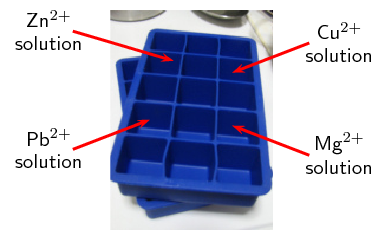2. Attach two different metals to the crocodile clips. Drape the wet string across the two solutions being used (so that each end of the string is in a solution). Then place the metal into its respective ion solution.

i.e. zinc electrode must go into the $$\text{Zn}^{2+}$$ solution, copper electrode must go into the $$\text{Cu}^{2+}$$ solution.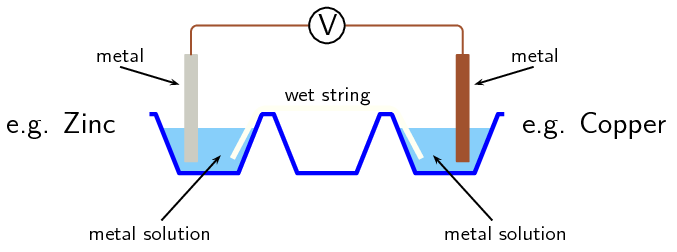Use the cell combinations in the following order:

Pb/Zn; Pb/Cu; Pb/Mg; Zn/Cu; Zn/Mg; Cu/Mg

3. Determine the combinations of metals that give a positive reading.

4. Draw up a table that shows:

• the combination of the metals

• which metal is the anode in that pair of metals

• which metal is the cathode in that pair of metals

 Metal combination Anode Cathode
5. Use this table to rank the metals from the strongest reducing agent (rank from the strongest to the weakest).

6. For each combination - write down the reduction half-reaction and oxidation half-reaction and then the overall cell reaction.

7. Note all observations for each cell.

### Questions

1. Explain why the voltages appear to be lower/higher than expected.

2. What is the purpose of the string?

### Conclusions

Depending on the electrode potential of each metal, the same metal could be the anode in one reaction and the cathode in another reaction. This can be seen by the positive, or negative, reading on the voltmeter.

For example, lead is more likely to be reduced than zinc, therefore in that pair lead will be the cathode and zinc will be the anode. However, lead is more likely to be oxidised than copper, therefore in that pair copper will be the cathode and lead will be the anode.

## Table of standard electrode potentials

Textbook Exercise 13.6

Give the standard electrode potential for each of the following metals:

magnesium

$$-\text{2,36}$$ $$\text{V}$$

$$-\text{0,13}$$ $$\text{V}$$

nickel

$$-\text{0,27}$$ $$\text{V}$$

Refer to the electrode potentials in Table 13.2.

Which of the metals is most likely to be oxidised?

lithium

Which metal ion is most likely to be reduced?

cobalt(III) (fluorine is not a metal)

Which metal is the strongest reducing agent?

lithium

If the other electrode is magnesium, is reduction or oxidation more likely to take place in the copper half-reaction? Explain your answer.

Reduction. The values given are for the reduction half-reactions. Copper is more easily reduced (postive electrode potential) than magnesium (negative electrode potential)

If the other electrode is tin, is reduction or oxidation more likely to take place in the mercury half-reaction? Explain your answer.

Reduction. The values given are for the reduction half-reactions. Mercury is more easily reduced (large, positive electrode potential) than tin (small, negative electrode potential).

Use the table of standard electrode potentials to put the following in order from the strongest oxidising agent to the weakest oxidising agent.

• $$\text{Cu}^{2+}$$

• $$\text{MnO}_{4}^{-}$$

• $$\text{Br}_{2}$$

• $$\text{Zn}^{2+}$$

The strongest oxidising agent will the compound that is most easily reduced. That is the the compound with the largest, positive E° value in the table of standard reduction potentials.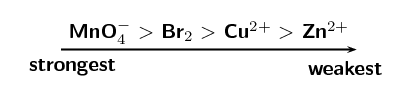Look at the following half-reactions:

• $$\text{Ca}^{2+}(\text{aq}) + 2\text{e}^{-}$$ $$\to$$ $$\text{Ca}(\text{s})$$
• $$\text{Cl}_{2}(\text{g}) + 2\text{e}^{-}$$ $$\to$$ $$2\text{Cl}^{-}(\text{aq})$$
• $$\text{Fe}^{3+}(\text{aq}) + 3\text{e}^{-}$$ $$\to$$ $$\text{Fe}(\text{s})$$
• $$\text{I}_{2}(\text{s}) + 2\text{e}^{-}$$ $$\to$$ $$2\text{I}^{-}(\text{aq})$$

Which substance is the strongest oxidising agent?

Chlorine is the strongest oxidising agent.

Which substance is the strongest reducing agent?

Calcium is the strongest reducing agent.

### Uses of the standard electrode potentials (ESCRK)

So now that you understand this useful table of reduction potentials, it is important that you can use these values to calculate the potential energy differences. The following worked examples will help you do this. In all of these cases it is important that you understand what the question is asking.

In this worked example you are given two half-reactions. Both are presented as shown in the table of standard reduction potentials, but in reality only one metal is being reduced, the other is being oxidised. The question being asked is: Which metal is being oxidised and which is being reduced?

## Worked example 7: Using the table of standard electrode potentials

The following reactions take place in an electrochemical cell:

$$\text{Cu}^{2+}(\text{aq}) + 2\text{e}^{-}$$ $$\rightleftharpoons$$ $$\text{Cu}(\text{s})$$

$$\text{Ag}^{+}(\text{aq}) + \text{e}^{-}$$ $$\rightleftharpoons$$ $$\text{Ag}(\text{s})$$

Determine which reaction is the oxidation half-reaction and which is the reduction half-reaction in this cell.

### Determine the electrode potential for each metal

From the table of standard electrode potentials:

$$\text{Cu}^{2+}(\text{aq}) + 2\text{e}^{-}$$ $$\rightleftharpoons$$ $$\text{Cu}(\text{s})$$ (E° = $$\text{+0,34}$$ $$\text{V}$$)

$$\text{Ag}^{+}(\text{aq}) + \text{e}^{-}$$ $$\rightleftharpoons$$ $$\text{Ag}(\text{s})$$ (E° = $$\text{+0,80}$$ $$\text{V}$$)

### Use the electrode potential values to determine which metal is oxidised and which is reduced

Both values are positive, but silver has a larger, positive electrode potential than copper. Therefore silver is more easily reduced than copper, and copper is more easily oxidised than silver.

### Write the reduction and oxidation half-reactions

The E° values are taken from the table of standard reduction potentials. Therefore the reduction half-reaction is as seen in the table.

The reduction half-reaction: $$\text{Ag}^{+}(\text{aq}) + \text{e}^{-}$$ $$\to$$ $$\text{Ag}(\text{s})$$ (reduction is a gain of electrons)

Oxidation is a loss of electrons, and so would be written in reverse.

The oxidation half-reaction: $$\text{Cu}(\text{s})$$ $$\to$$ $$\text{Cu}^{2+}(\text{aq}) + 2\text{e}^{-}$$.

## Worked example 8: Using the table of standard electrode potentials

Is magnesium able to displace silver from a solution of silver nitrate?

### Find appropriate reactions on the table of standard electrode potentials

The reaction involves magnesium and silver.

$$\text{Mg}^{2+}(\text{aq}) + 2\text{e}^{-}$$ $$\rightleftharpoons$$ $$\text{Mg}(\text{s})$$

$$\text{Ag}^{+}(\text{aq}) + \text{e}^{-}$$ $$\rightleftharpoons$$ $$\text{Ag}(\text{s})$$

### Determine the electrode potential for each metal

From the table of standard electrode potentials:

$$\text{Mg}^{2+}(\text{aq}) + 2\text{e}^{-}$$ $$\rightleftharpoons$$ $$\text{Mg}(\text{s})$$ (E° = $$-\text{2,36}$$ $$\text{V}$$)

$$\text{Ag}^{+}(\text{aq}) + \text{e}^{-}$$ $$\rightleftharpoons$$ $$\text{Ag}(\text{s})$$ (E° = $$\text{+0,80}$$ $$\text{V}$$)

### Which metal is more likely to be reduced, which is more likely to be oxidised?

Silver has a positive E°, while magnesium has a negative E°. Therefore silver is more easily reduced than magnesium, and magnesium is more easily oxidised than silver. The following reactions would occur:

Reduction half-reaction: $$\text{Ag}^{+}(\text{aq}) + \text{e}^{-}$$ $$\to$$ $$\text{Ag}(\text{s})$$

Oxidation half-reaction: $$\text{Mg}(\text{s})$$ $$\to$$ $$\text{Mg}^{2+}(\text{aq}) + 2\text{e}^{-}$$

It can be concluded that magnesium will displace silver from a silver nitrate solution so that there will be silver metal and magnesium ions in the solution.

If magnesium is able to displace silver from a solution of silver nitrate, this means that magnesium metal will form magnesium ions and the silver ions will become silver metal. In other words, there will now be silver metal and a solution of magnesium nitrate. This will only happen if magnesium has a greater tendency than silver to form ions. In other words, what this worked example is asking is whether magnesium or silver will form ions more easily.

Remember that solid metal will not always be formed when an ion is reduced. For example $$\text{Sn}^{4+} + 2\text{e}^{-}$$ $$\to$$ $$\text{Sn}^{2+}$$.

## Worked example 9: Determining overall reactions

For a zinc ($$\text{Zn}$$) and gold(III) oxide ($$\text{Au}_{2}\text{O}_{3}$$) cell in solution of $$\text{KOH}$$ determine the:

• oxidation and reduction half-reactions

• overall balanced chemical equation

• standard cell notation for the cell

### Find appropriate reactions on the table of standard electrode potentials

The reaction involves zinc and gold.

$$\text{Zn}^{2+}(\text{aq}) + 2\text{e}^{-}$$ $$\rightleftharpoons$$ $$\text{Zn}(\text{s})$$

$$\text{Au}^{3+}(\text{aq}) + 3\text{e}^{-}$$ $$\rightleftharpoons$$ $$\text{Au}(\text{s})$$

### Determine the electrode potential for each metal

From the table of standard electrode potentials:

$$\text{Zn}^{2+}(\text{aq}) + 2\text{e}^{-}$$ $$\rightleftharpoons$$ $$\text{Zn}(\text{s})$$ (E° = $$-\text{0,76}$$ $$\text{V}$$)

$$\text{Au}^{3+}(\text{aq}) + 3\text{e}^{-}$$ $$\rightleftharpoons$$ $$\text{Au}(\text{s})$$ (E° = $$\text{+1,52}$$ $$\text{V}$$)

### Which metal is more likely to be reduced, which is more likely to be oxidised?

Zinc has a negative E°, while gold has a positive E°. Therefore gold is more easily reduced than zinc, and zinc is more easily oxidised than gold. The following reactions would occur:

Reduction half-reaction: $$\text{Au}^{3+}(\text{aq}) + 3\text{e}^{-}$$ $$\to$$ $$\text{Au}(\text{s})$$

Oxidation half-reaction: $$\text{Zn}(\text{s})$$ $$\to$$ $$\text{Zn}^{2+}(\text{aq}) + 2\text{e}^{-}$$

### Compare the number of electrons in each equation

There are $$\text{3}$$ electrons in the reduction half-reaction, and $$\text{2}$$ electrons in the oxidation half-reaction.

Oxidation: $$\color{red}{\times 3}$$: $$\phantom{\rule{6.pt}{0ex}}$$ $$\color{red}{3}$$$$\text{Zn}(\text{s})$$ $$\to \color{red}{3}$$$$\text{Zn}^{2+}(\text{aq}) +$$ $$\color{red}{\textbf{6}}\textbf{e}^{-}$$

Reduction: $$\color{red}{\times 2}$$: $$\color{red}{2}$$$$\text{Au}^{3+}(\text{aq}) +$$ $$\color{red}{\textbf{6}}\textbf{e}^{-} \to \color{red}{2}$$$$\text{Au}(\text{s})$$

### Combine the equations into one equation

$$2\text{Au}^{3+}(\text{aq}) + 6\text{e}^{-} + 3\text{Zn}(\text{s})$$ $$\to$$ $$2\text{Au}(\text{s}) + 3\text{Zn}^{2+}(\text{aq}) + 6\text{e}^{-}$$

### Add the spectator ions and remove the electrons from the equation

$$\text{Au}_{2}\text{O}_{3}(\text{aq}) + 3\text{Zn}(\text{s})$$ $$\to$$ $$2\text{Au}(\text{s}) + 3\text{ZnO}(\text{aq})$$

### Which material is the anode and which is the cathode?

Oxidation is loss of electrons at the anode, therefore $$\text{Zn}(\text{s})$$ is the anode.

Reduction is gain of electrons at the cathode, therefore $$\text{Au}_{2}\text{O}_{3}$$ is the cathode.

### Give the standard cell notation for this reaction

The anode is always written first (on the left): $$\text{Zn}(\text{s})|\text{ZnO}(\text{aq})$$

The cathode is always written second (on the right): $$\text{Au}_{2}\text{O}_{3}(\text{s}),\text{Au}(\text{s})$$

Therefore the standard cell notation is:

$$\text{Zn}(\text{s})|\text{ZnO}(\text{aq})||\text{Au}_{2}\text{O}_{3}(\text{s}),\text{Au}(\text{s})$$

In the displacement experiment you can use xylene, toluene or carbon disulfide instead of paraffin. These are not great chemicals to work with however. If you do use one of these chemicals make sure the learners research the hazards of the materials they are working with.

Learners are required to work with a concentrated, strong acid. Concentrated, strong acids can cause serious burns. Please remind the learners to be careful and wear the appropriate safety equipment when handling all chemicals, especially concentrated acids. The safety equipment includes gloves, safety glasses and protective clothing.

## Displacement experiment

### Aim

To demonstrate the effect of reacting a halogen with a halide.

### Apparatus

• Bleach (approximately $$\text{10}$$ $$\text{cm^{3}}$$), bromine water, aqueous solutions of sodium chloride ($$\text{NaCl}$$), sodium bromide ($$\text{NaBr}$$) and sodium iodide ($$\text{NaI}$$), paraffin, concentrated $$\text{HCl}$$.

• 5 test tubes, 2 plastic droppers.

### Method

Concentrated $$\text{HCl}$$ can cause serious burns. We suggest using gloves and safety glasses whenever you work with an acid. Remember to add the acid to the water and to avoid sniffing the acid. Handle all chemicals with care.

1. Label the test tubes A, B, C, D and E.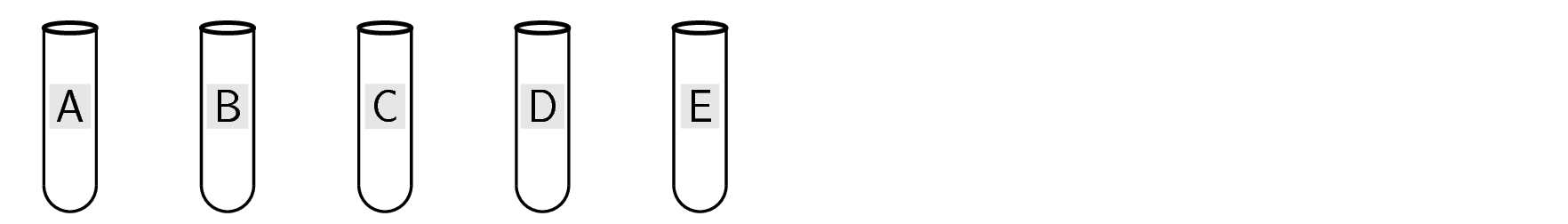2. Place $$\text{1}$$ $$\text{cm^{3}}$$ $$\text{NaCl}$$ solution into test tube A.

3. Place $$\text{1}$$ $$\text{cm^{3}}$$ $$\text{NaBr}$$ solution into both test tubes B and C.

4. Place $$\text{1}$$ $$\text{cm^{3}}$$ $$\text{NaI}$$ solution into both test tubes D and E.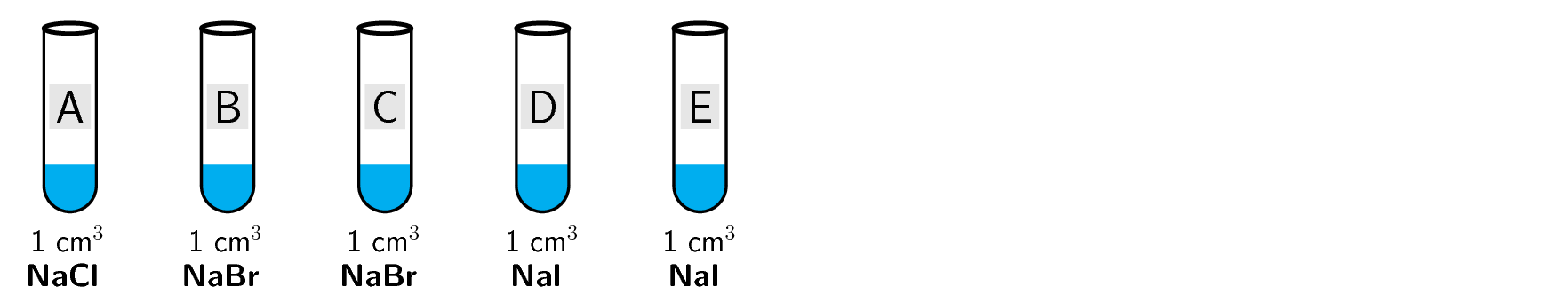5. Activate $$\text{10}$$ $$\text{cm^{3}}$$ of the bleach by adding $$\text{2}$$ $$\text{cm^{3}}$$ of the concentrated $$\text{HCl}$$.

Observe the liquid and note what happens on adding $$\text{HCl}$$, record your observations. You have formed a solution of chlorine in water.

6. Using a plastic dropper transfer approximately $$\text{1}$$ $$\text{cm}$$ height of the chlorine water into the test tubes labelled A, B and D.

Note any changes to the test tube. Record all observations.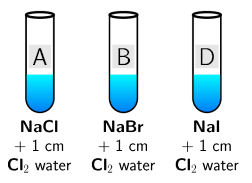7. Pour $$\text{1}$$ $$\text{cm^{3}}$$ of bromine water into the test tubes labelled C and E.

Note any changes to the test tube. Record all observations.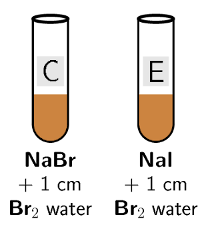8. Using a plastic dropper transfer approximately $$\text{2}$$ $$\text{cm}$$ height of the paraffin into each test tube. Use a cork or rubber stopper to close the test tube, hold it firmly in place with your thumb, and shake the mixture.9. Use the redox table to write overall net ionic equations for the reactions in test tubes B, D and E.

10. Using your understanding of solubility rules (like dissolves like) explain why the layer of paraffin became coloured in test tubes B, D and E. Explain what caused the paraffin to become coloured in test tube C.

11. Why was there no reaction and no colour change in test tube A?

### Results

• In test tube A $$\text{Cl}_{2}$$ is present, but is not coloured and so no change in the colour of the paraffin is observed.

• In test tubes B and D the chlorine displaces the $$\text{Br}^{-}$$ and $$\text{I}^{-}$$ ions. $$\text{Br}_{2}$$ and $$\text{I}_{2}$$ are formed.

$$\text{Br}_{2}$$ is a brown colour in paraffin, while $$\text{I}_{2}$$ is a purple colour in paraffin.

• In test tube C the $$\text{Br}_{2}$$ was already present and coloured the paraffin a brown colour.

• In test tube E the $$\text{Br}_{2}$$ displaced the $$\text{I}^{-}$$ ions to form $$\text{I}_{2}$$ which would turn the paraffin a purple colour.

### Conclusion

Halogen molecules are non-polar. They will therefore dissolve in a non-polar solvent such as paraffin. The paraffin layer will become the colour of the halogen present in the solution. Chlorine is the most likely of these three halogens to be reduced, followed by bromine, and then iodine. This can be seen on the standard electrode potential table as chlorine has the largest, positive electrode potential of the three halogens.

## Using standard electrode potentials

Textbook Exercise 13.7

If silver was added to a solution of copper(II) sulfate, would it displace the copper from the copper(II) sulfate solution? Explain your answer.

No.

We use the table of standard electrode potentials to find the electrode potential for silver and for copper.

Silver: $$\text{Ag}^{+}(\text{aq}) + \text{e}^{-}$$ $$\rightleftharpoons$$ $$\text{Ag}(\text{s})$$ (E° = $$\text{+0,80}$$ $$\text{V}$$)

Copper: $$\text{Cu}^{2+}(\text{aq}) + 2\text{e}^{-}$$ $$\rightleftharpoons$$ $$\text{Cu}(\text{s})$$ (E° = $$\text{+0,34}$$ $$\text{V}$$)

Silver has a larger, positive E° than copper. Therefore silver is more easily reduced (more easily forms solid metal) than copper (more easily forms copper ions) and will not be able to displace the copper ions from the solution.

If zinc is added to a solution of magnesium sulfate, will the zinc displace the magnesium from the solution? Give a detailed explanation for your answer.

No.

We use the table of standard electrode potentials to find the electrode potential for zinc and for magnesium.

Zinc: $$\text{Zn}^{2+}(\text{aq}) + 2\text{e}^{-}$$ $$\rightleftharpoons$$ $$\text{Zn}(\text{s})$$ (E° = $$-\text{0,76}$$ $$\text{V}$$)

Magnesium: $$\text{Mg}^{2+}(\text{aq}) + 2\text{e}^{-}$$ $$\rightleftharpoons$$ $$\text{Mg}(\text{s})$$ (E° = $$-\text{2,36}$$ $$\text{V}$$)

Zinc has a smaller, negative E° than magnesium. Therefore zinc is more easily reduced (more easily forms solid metal) than magnesium (more easily forms magnesium ions) and will not be able to displace the magnesium ions from the solution.

If aluminium is added to a solution of cobalt sulfate, will the aluminium displace the cobalt from the solution? Give a detailed explanation for your answer.

Yes.

We use the table of standard electrode potentials to find the electrode potential for aluminium and for cobalt.

Aluminium: $$\text{Al}^{3+}(\text{aq}) + 3\text{e}^{-}$$ $$\rightleftharpoons$$ $$\text{Al}(\text{s})$$ (E° = $$-\text{1,66}$$ $$\text{V}$$)

Cobalt: $$\text{Co}^{2+}(\text{aq}) + 2\text{e}^{-}$$ $$\rightleftharpoons$$ $$\text{Co}(\text{s})$$ (E° = $$-\text{0,28}$$ $$\text{V}$$)

Aluminium has a larger, negative E° than cobalt. Therefore aluminium is more easily oxidised (more easily forms aluminium ions) than cobalt (more easily forms solid metal) and will be able to displace the cobalt ions from the solution.

### EMF of a cell (ESCRM)

Using the example of the zinc and copper half-cells, we know that when these two half-cells are combined, zinc will be the oxidation half-reaction and copper will be the reduction half-reaction. A voltmeter connected to this cell will show that the zinc electrode is more negative than the copper electrode.

$$\color{red}{\text{Cu}^{2+}\text{(aq) + 2e}^{-} \rightleftharpoons \text{Cu(s)}}$$ (E° = $$\text{+0,34}$$ $$\text{V}$$)

$$\color{blue}{\text{Zn}^{2+}\text{(aq) + 2e}^{-} \rightleftharpoons \text{Zn(s)}}$$ (E° = $$-\text{0,76}$$ $$\text{V}$$)

The reading on the meter will show the potential difference between the two half-cells. This is known as the EMF of the cell. The higher the EMF, the greater amount of energy released per unit charge.

EMF of a cell

The EMF of a cell is defined as the maximum potential difference between two electrodes or half-cells in a galvanic cell.

The EMF of a cell is a the same as the voltage across a disconnected cell (electric circuit theory). A voltmeter is effectively a high resistance ammeter, so a very small current will flow when a voltmeter reading is taken (although this is too small to be noticeable).

Remember:

Standard conditions are:

p = $$\text{101,3}$$ $$\text{kPa}$$

C = $$\text{1}$$ $$\text{mol·dm^{-3}}$$

T = $$\text{298}$$ $$\text{K}$$.

$$\_\_\_\_\_\_\_\_\_\_\_\_\_\_$$

In standard cell notation the $$\color{blue}{\textbf{anode half-cell}}$$ is always written on the $$\color{blue}{\text{left}}$$ and the $$\color{red}{\textbf{cathode half-cell}}$$ is always written on the $$\color{red}{\text{right}}$$.

$$\_\_\_\_\_\_\_\_\_\_\_\_\_\_$$

The $$\color{blue}{\text{reducing agent}}$$ is being $$\color{blue}{\text{oxidised}}$$. $$\color{blue}{\text{Oxidation}}$$ is a loss of electrons at the $$\color{blue}{\text{anode}}$$.The $$\color{red}{\text{oxidising agent}}$$ is being $$\color{red}{\text{reduced}}$$. $$\color{red}{\text{Reduction}}$$ is a gain of electrons at the $$\color{red}{\text{cathode}}$$.It is important to be able to calculate the EMF of an electrochemical cell. To calculate the EMF of a cell:

• take the E° of the atom that is being $$\color{red}{\text{reduced}}$$

• subtract the E° of the atom that is being $$\color{blue}{\text{oxidised}}$$

The reason for defining a reference electrode becomes obvious now. Potential differences can be calculated from the electrode potentials (determined relative to the hydrogen half-cell) without having to construct the cells themselves each time.

You can use any one of the following equations:

• E°$$_{\text{(cell)}}=$$ E°($$\color{red}{\text{reduction half-reaction}}$$) - E°($$\color{blue}{\text{oxidation half-reaction}}$$)

• E°$$_{\text{(cell)}}=$$ E°($$\color{red}{\text{oxidising agent}}$$) - E°($$\color{blue}{\text{reducing agent}}$$)

• E°$$_{\text{(cell)}}=$$ E°($$\color{red}{\text{cathode}}$$) - E°($$\color{blue}{\text{anode}}$$)

So, for the $$\text{Zn}$$-$$\text{Cu}$$ cell:

E°$$_{\text{(cell)}} =$$ $$\text{0,34} - (-\text{0,76}$$) = $$\text{0,34}$$ + $$\text{0,76}$$ = $$\text{1,10}$$ $$\text{V}$$

Standard EMF

Standard EMF (E°$$_{\text{cell}}$$) is the EMF of a galvanic cell operating under standard conditions. The symbol ° denotes standard conditions.

## Worked example 10: Calculating the EMF of a cell

A cell contains a solid lead anode in a gold ion solution.

1. Represent the cell using standard notation.

2. Calculate the cell potential (EMF) of the electrochemical cell.

### Find appropriate reactions on the table of standard electrode potentials

The reaction involves lead and gold.

$$\text{Pb}^{2+}(\text{aq}) + 2\text{e}^{-}$$ $$\rightleftharpoons$$ $$\text{Pb}(\text{s})$$ (E° V = $$-\text{0,13}$$ $$\text{V}$$)

$$\text{Au}^{3+}(\text{aq}) + 3\text{e}^{-}$$ $$\rightleftharpoons$$ $$\text{Au}(\text{s})$$ (E° V = $$\text{+1,52}$$ $$\text{V}$$)

### Which metal is more likely to be reduced, which is more likely to be oxidised?

The E° of lead is a small, negative value and the E° of gold is a large, positive number. Therefore lead is more easily oxidised than gold, and gold is more easily reduced than lead.

### Determine which metal is the cathode and which is the anode

Oxidation is loss at the anode, therefore lead is the anode.

Reduction is gain at the cathode, therefore gold is the cathode.

### Represent the cell using standard cell notation

The anode is always written on the left, the cathode on the right.

$$\text{Pb}(\text{s})|\text{Pb}^{2+}(\text{aq})||\text{Au}^{3+}(\text{aq})|\text{Au}(\text{s})$$

### Calculate the cell potential

E°$$_{\text{(cell)}}=$$ E°$$_\text{(cathode)}$$ - E°$$_\text{(anode)}$$

E°$$_{\text{(cell)}}=$$ E°$$_\text{(gold)}$$ - E°$$_\text{(lead)}$$

= $$\text{+1,52} - (-\text{0,13}$$)

= $$\text{+1,65}$$ $$\text{V}$$

## Worked example 11: Calculating the EMF of a cell

Calculate the cell potential of the electrochemical cell in which the following reaction takes place, and represent the cell using standard notation.

$$\text{Mg}(\text{s}) + 2\text{H}^{+}(\text{aq})$$ $$\to$$ $$\text{Mg}^{2+}(\text{aq}) + \text{H}_{2}(\text{g})$$

### Find appropriate reactions on the table of standard electrode potentials

$$\text{Mg}^{2+}(\text{aq}) + 2\text{e}^{-}$$ $$\rightleftharpoons$$ $$\text{Mg}(\text{s})$$ (E° = $$-\text{2,36}$$ $$\text{V}$$)

$$2\text{H}^{+}(\text{aq}) + 2\text{e}^{-}$$ $$\rightleftharpoons$$ $$\text{H}_{2}(\text{g})$$ (E° = $$\text{0,00}$$ $$\text{V}$$)

### Which element is more likely to be reduced, which is more likely to be oxidised?

The E° of magnesium is a more negative value than the E° of hydrogen. Therefore magnesium is more easily oxidised than hydrogen, and hydrogen is more easily reduced than magnesium.

### Determine which metal is the cathode and which is the anode

Oxidation is loss at the anode, therefore magnesium is the anode.

Reduction is gain at the cathode, therefore hydrogen is the cathode reaction.

### Represent the cell using standard notation

The anode is always written on the left, the cathode on the right. The hydrogen electrode includes an inert platinum plate.

$$\text{Mg}(\text{s})|\text{Mg}^{2+}(\text{aq})||\text{H}^{+}(\text{aq}),\text{H}_{2}(\text{g})|\text{Pt}(\text{s})$$

### Calculate the cell potential

E$$_{\text{(cell)}} =$$ E°$$_\text{(cathode)}$$ - E°$$_\text{(anode)}$$

E$$_{\text{(cell)}} =$$ E°$$_\text{(hydrogen)}$$ - E°$$_\text{(magnesium)}$$

= $$\text{0,00} - (-\text{2,37}$$)

=$$\text{+2,36}$$ $$\text{V}$$

temp text

## Standard electrode potentials

Textbook Exercise 13.8

In your own words, explain what is meant by the 'electrode potential' of a metal.

Electrode potential of a metal is the: 'EMF of a cell in which the electrode on the left is a standard hydrogen electrode and the electrode on the right is the electrode in question' - from the IUPAC Gold Book (goldbook.iupac.org)

That is, the standard electrode potential for a metal was measured in a cell where there was a standard hydrogen electrode on the left, and the metal being studied on the right. The EMF measured for that cell is the standard electrode potential of that metal. The positive or negative value obtained depends on whether the metal is more, or less easily reduced than hydrogen.

Calculate the EMF for each of the following standard electrochemical cells:

$$\text{Mn}(\text{s})|\text{Mn}^{2+}(\text{aq})||\text{H}^{+}(\text{aq}),\text{H}_{2}(\text{g})|\text{Pt}(\text{s})$$

The anode is written on the left, the cathode is written on the right.

$$\text{Mn}^{2+}(\text{aq}) + 2\text{e}^{-}$$ $$\rightleftharpoons$$ $$\text{Mn}(\text{s})$$ (E° = $$-\text{1,18}$$ $$\text{V}$$)

$$2\text{H}^{+}(\text{aq}) + 2\text{e}^{-}$$ $$\rightleftharpoons$$ $$\text{H}_{2}(\text{g})$$ (E° = $$\text{0,00}$$ $$\text{V}$$)

E°$$_\text{(cell)}$$ = E°$$_\text{(cathode)}$$ - E°$$_\text{(anode)}$$

E°$$_\text{(cell)}$$ = E°$$_\text{(hydrogen)}$$ - E°$$_\text{(manganese)}$$ = $$\text{0}$$ - ($$-\text{1,18}$$) = $$\text{+1,18}$$ $$\text{V}$$

$$\text{Fe}(\text{s})|\text{Fe}^{3+}(\text{aq})||\text{Fe}^{2+}(\text{aq})|\text{Fe}(\text{s})$$

The anode is written on the left, the cathode is written on the right.

$$\text{Fe}^{3+}(\text{aq}) + 3\text{e}^{-}$$ $$\rightleftharpoons$$ $$\text{Fe}(\text{s})$$ (E° = $$-\text{0,06}$$ $$\text{V}$$)

$$\text{Fe}^{2+}(\text{aq}) + 2\text{e}^{-}$$ $$\rightleftharpoons$$ $$\text{Fe}(\text{s})$$ (E° = $$-\text{0,44}$$ $$\text{V}$$)

E°$$_\text{(cell)}$$ = E°$$_\text{(cathode)}$$ - E°$$_\text{(anode)}$$

E°$$_\text{(cell)}$$ = E°$$_\text{(iron(II))}$$ - E°$$_\text{(iron(III))}$$ = $$-\text{0,44} - (-\text{0,06}$$) = $$-\text{0,38}$$ $$\text{V}$$

$$\text{Cr}(\text{s})|\text{Cr}^{2+}(\text{aq})||\text{Cu}^{2+}(\text{aq})|\text{Cu}(\text{s})$$

The anode is written on the left, the cathode is written on the right.

$$\text{Cr}^{2+}(\text{aq}) + 2\text{e}^{-}$$ $$\rightleftharpoons$$ $$\text{Cr}(\text{s})$$ (E° = $$-\text{0,74}$$ $$\text{V}$$)

$$\text{Cu}^{2+}(\text{aq}) + 2\text{e}^{-}$$ $$\rightleftharpoons$$ $$\text{Cu}(\text{s})$$ (E° = $$\text{+0,34}$$ $$\text{V}$$)

E°$$_\text{(cell)}$$ = E°$$_\text{(cathode)}$$ - E°$$_\text{(anode)}$$

E°$$_\text{(cell)}$$ = E°$$_\text{(copper)}$$ - E°$$_\text{(chromium)}$$ = $$\text{+0,34}$$ - ($$-\text{0,74}$$) = $$\text{+1,08}$$ $$\text{V}$$

$$\text{Pb}(\text{s})|\text{Pb}^{2+}(\text{aq})||\text{Hg}^{2+}(\text{aq})|\text{Hg}(\text{ℓ})$$

The anode is written on the left, the cathode is written on the right.

$$\text{Pb}^{2+}(\text{aq}) + 2\text{e}^{-}$$ $$\rightleftharpoons$$ $$\text{Pb}(\text{s})$$ (E° = $$\text{+0,13}$$ $$\text{V}$$)

$$\text{Hg}^{2+}(\text{aq}) + 2\text{e}^{-}$$ $$\rightleftharpoons$$ $$\text{Hg}(\text{ℓ})$$ (E° = $$\text{+0,85}$$ $$\text{V}$$)

E°$$_\text{(cell)}$$ = E°$$_\text{(cathode)}$$ - E°$$_\text{(anode)}$$

E°$$_\text{(cell)}$$ = E°$$_\text{(mercury)}$$ - E°$$_\text{(lead)}$$ = $$\text{+0,85} - (-\text{0,13}$$) = $$\text{+0,98}$$ $$\text{V}$$

Given the following two half-reactions:

$$\text{Fe}^{3+}(\text{aq}) + \text{e}^{-}$$ $$\rightleftharpoons$$ $$\text{Fe}^{2+}(\text{aq})$$

$$\text{MnO}_{4}^{-}(\text{aq}) + 8\text{H}^{+}(\text{aq}) + 5\text{e}^{-}$$ $$\rightleftharpoons$$ $$\text{Mn}^{2+}(\text{aq}) + 4\text{H}_{2}\text{O}(\text{ℓ})$$

Give the standard electrode potential for each half-reaction.

$$\text{Fe}^{3+}(\text{aq}) + \text{e}^{-}$$ $$\rightleftharpoons$$ $$\text{Fe}^{2+}(\text{aq})$$ (E° = $$\text{+0,77}$$ $$\text{V}$$)

$$\text{MnO}_{4}^{-}(\text{aq}) + 8\text{H}^{+}(\text{aq}) + 5\text{e}^{-}$$ $$\rightleftharpoons$$ $$\text{Mn}^{2+}(\text{aq}) + 4\text{H}_{2}\text{O}(\text{ℓ})$$ (E° = $$\text{+1,51}$$ $$\text{V}$$)

Which reaction takes place at the cathode and which reaction takes place at the anode?

The permanganate ion has a larger, positive E° than an iron(III) ion. Therefore permanganate is more easily reduced. Reduction occurs at the cathode, therefore the permanganate ion is the cathode.

The iron ion is more easily oxidised. Oxidation occurs at the anode, therefore iron(II) ion is the anode.

Represent the electrochemical cell using standard notation (the cathode and anode reactions take place at inert platinum electrodes).

$$\text{Pt}(\text{s})|\text{Fe}^{2+}(\text{aq}),\text{Fe}^{3+}(\text{aq})||\text{MnO}_{4}^{-}(\text{aq}),\text{Mn}^{2+}(\text{aq})|\text{Pt}(\text{s})$$

Calculate the EMF of the cell

E°$$_\text{(cell)}$$ = E°$$_\text{(cathode)}$$ - E°$$_\text{(anode)}$$

E°$$_\text{(cell)}$$ = E°$$_\text{(permanganate ion)}$$ - E°$$_\text{(iron ion)}$$= $$\text{1,52} - \text{0,77} = \text{+0,75} \text{ V}$$

### Spontaneity (ESCRN)

 Spontaneous positive EMF Non-spontaneous negative EMF

Table 13.4: Using EMF to determine cell spontaneity.

You can see from the table of reduction potentials (Table 13.2) that different metals have different reactivities. Some are reduced more easily than others. You can also say that some are oxidised more easily than others.

For example, copper (E° = $$\text{+0,34}$$ $$\text{V}$$) is more easily reduced than zinc (E° = $$-\text{0,76}$$ $$\text{V}$$). Therefore, if a reaction involves the reduction of copper and the oxidation of zinc, it will occur spontaneously. If, however, it requires the oxidation of copper and the reduction of zinc, it will not occur spontaneously.

To predict whether a reaction occurs spontaneously you can look at the sign of the EMF value for the cell. If the EMF is positive then the reaction is spontaneous. If the EMF is negative then the reaction is not spontaneous.

One can perform experiments to predict whether a reaction will be spontaneous or not. It turns out that the sign of the EMF is equivalent to whether a cell reaction is spontaneous or not. Those reactions that are spontaneous have a positive EMF and those reactions that are non-spontaneous have a negative EMF.

Look at the following example to help you to understand how to predict whether a reaction will take place spontaneously or not.

In the reaction: $$\color{red}{\text{Pb}^{2+}{\text{(aq)}}} + \color{blue}{\text{2Br}^{-}{\text{(aq)}}} \rightleftharpoons \color{blue}{\text{Br}_{2}{\text{(l)}}} + \color{red}{\text{Pb(s)}}$$

the two half-reactions are as follows:

$$\color{red}{\text{Pb}^{2+}{\text{(aq)}} + {\text{2e}}^{-} \rightleftharpoons \text{Pb(s)}}$$ (E° = $$-\text{0,13}$$ $$\text{V}$$)

$$\color{blue}{\text{Br}_{2}{\text{(l)}} + {\text{2e}}^{-} \rightleftharpoons \text{2Br}^{-}{\text{(aq)}}}$$ (E° = $$\text{+1,07}$$ $$\text{V}$$)

EMF = E°(reduction half-reaction) - E°(oxidation half-reaction)

EMF = $$-\text{0,13}$$ $$\text{V} - \text{1,07}$$ = $$-\text{1,2}$$ $$\text{V}$$

The sign of the EMF is negative, therefore this reaction will not take place spontaneously. Let's look at the reasoning behind this in more detail.

Look at the electrode potential for the first half-reaction. The negative value shows that lead loses electrons more easily than bromine, in other words it is easily oxidised. However, in the original equation, lead ions ($$\text{Pb}^{2+}$$) are being reduced. This part of the reaction is not spontaneous.

For the second half-reaction the positive electrode potential value shows that bromine is more easily reduced than lead: bromine will more easily gain electrons to become $$\text{Br}^{-}$$. This is not what is happening in the original equation and therefore this is also not spontaneous.

It therefore makes sense that the reaction will not proceed spontaneously.

## Worked example 12: Determining spontaneity

Will copper react with dilute sulfuric acid ($$\text{H}_{2}\text{SO}_{4}$$)? You are given the following half-reactions:

$$\text{Cu}^{2+}(\text{aq}) + 2\text{e}^{-}$$ $$\rightleftharpoons$$ $$\text{Cu}(\text{s})$$ (E° = $$\text{+0,34}$$ $$\text{V}$$)

$$2\text{H}^{+}(\text{aq}) + 2\text{e}^{-}$$ $$\rightleftharpoons$$ $$\text{H}_{2}(\text{g})$$ (E° = $$\text{0,00}$$ $$\text{V}$$)

### Determine the overall equation for this reaction

The question asked if copper metal will react with dilute sulfuric acid, therefore the reactants are $$\text{Cu}(\text{s})$$ and $$\text{H}^{+}(\text{aq})$$:

$$\text{Cu}(\text{s}) + 2\text{H}^{+}(\text{aq})$$ $$\to$$ $$\text{Cu}^{2+}(\text{aq}) + \text{H}_{2}(\text{g})$$

### Which reactant should be oxidised, and which should be reduced?

$$\text{Cu}(\text{s})$$ $$\to$$ $$\text{Cu}^{2+}(\text{aq}) + 2\text{e}^{-}$$, therefore copper needs to be oxidised.

$$2\text{H}^{+}(\text{aq}) + 2\text{e}^{-}$$ $$\to$$ $$\text{H}_{2}(\text{g})$$, therefore hydrogen ions needs to be reduced.

### Predict whether the reaction will be spontaneous or non-spontaneous

Copper has a larger, positive E° than hydrogen. Therefore copper is more easily reduced than hydrogen, and hydrogen is more easily oxidised than copper.

The reaction is non-spontaneous.

### Calculate the EMF of the cell

E$$^{\circ}_{\text{(cell)}}$$ = E$$_{\text{(reduction)}}$$ - E$$_{\text{(oxidation)}}$$

E$$^{\circ}_{\text{(cell)}}$$ = E$$_{\text{(hydrogen ions)}}$$ - E$$_{\text{(copper)}}$$

E$$^{\circ}_{\text{(cell)}}$$ = $$\text{0,00}$$ $$\text{V}$$ - ($$\text{+0,34}$$ $$\text{V}$$) = $$-\text{0,34}$$ $$\text{V}$$

### Is the reaction spontaneous?

The EMF is negative, therefore the reaction is non-spontaneous.

## Worked example 13: Determining spontaneity

Will zinc react with dilute hydrochloric acid ($$\text{HCl}$$)? You are given the following half-reactions:

$$\text{Zn}^{2+}(\text{aq}) + 2\text{e}^{-}$$ $$\rightleftharpoons$$ $$\text{Zn}(\text{s})$$ (E° = $$-\text{0,76}$$ $$\text{V}$$)

$$2\text{H}^{+}(\text{aq}) + 2\text{e}^{-}$$ $$\rightleftharpoons$$ $$\text{H}_{2}(\text{g})$$ (E° = $$\text{0,00}$$ $$\text{V}$$)

### Determine the overall equation for this reaction

The question asked if zinc metal will react with dilute hydrochloric acid, therefore the reactants are $$\text{Zn}(\text{s})$$ and $$\text{H}^{+}$$:

$$\text{Zn}(\text{s}) + 2\text{H}^{+}(\text{aq})$$ $$\to$$ $$\text{Zn}^{2+}(\text{aq}) + \text{H}_{2}(\text{g})$$

### Which reactant should be oxidised, and which should be reduced?

$$\text{Zn}(\text{s})$$ $$\to$$ $$\text{Zn}^{2+}(\text{aq}) + 2\text{e}^{-}$$, therefore zinc needs to be oxidised.

$$2\text{H}^{+}(\text{aq}) + 2\text{e}^{-}$$ $$\to$$ $$\text{H}_{2}(\text{g})$$, therefore hydrogen ions need to be reduced.

### Predict whether the reaction will be spontaneous or non-spontaneous

Zinc has a larger, negative E° than hydrogen. Therefore zinc is more easily oxidised than hydrogen, and hydrogen is more easily reduced than copper.

The reaction is spontaneous.

### Calculate the EMF of the cell

E$$^{\circ}_{\text{(cell)}}$$ = E$$_{\text{(reduction)}}$$ - E$$_{\text{(oxidation)}}$$

E$$^{\circ}_{\text{(cell)}}$$ = E$$_{\text{(hydrogen ions)}}$$ - E$$_{\text{(zinc)}}$$

E$$^{\circ}_{\text{(cell)}}$$ = $$\text{0,00}$$ $$\text{V}$$ - ($$-\text{0,76}$$ $$\text{V}$$) = $$\text{+0,76}$$ $$\text{V}$$

### Is the reaction spontaneous?

The EMF is positive, therefore the reaction is spontaneous.

temp text

## Predicting spontaneity

Textbook Exercise 13.9

Will the following reaction take place spontaneously or not? Show all your working.

$$2\text{Ag}(\text{s}) + \text{Cu}^{2+}(\text{aq})$$ $$\to$$ $$\text{Cu}(\text{s}) + 2\text{Ag}^{+}(\text{aq})$$

$$\text{Ag}^{+}(\text{aq}) + \text{e}^{-}$$ $$\rightleftharpoons$$ $$\text{Ag}(\text{s})$$ (E° = $$\text{+0,80}$$ $$\text{V}$$)

$$\text{Cu}^{2+}(\text{aq}) + 2\text{e}^{-}$$ $$\rightleftharpoons$$ $$\text{Cu}(\text{s})$$ (E° = $$\text{+0,34}$$ $$\text{V}$$)

In the balanced chemical equation solid Ag is oxidised and copper ions are reduced:

Oxidation half-reaction: $$\text{Ag}(\text{s})$$ $$\to$$ $$\text{Ag}^{+}(\text{aq}) + \text{e}^{-}$$

Reduction half-reaction: $$\text{Cu}^{2+}(\text{aq}) + 2\text{e}^{-}$$ $$\to$$ $$\text{Cu}(\text{s})$$

E°$$_{\text{(cell)}}$$ = E°$$_{\text{(reduction)}}$$ - E°$$_{\text{(oxidation)}}$$

E°$$_{\text{(cell)}}$$ = E°$$_{\text{(copper)}}$$ - E°$$_{\text{(silver)}}$$

E°$$_{\text{(cell)}}$$ = $$\text{+0,34}$$ $$\text{V}$$ - ($$\text{+0,80}$$ $$\text{V}$$) = $$-\text{0,46}$$ $$\text{V}$$

The E°$$_{\text{(cell)}}$$ is negative, therefore this reaction is not spontaneous.

Nickel metal reacts with an acid, $$\text{H}^{+}$$ (aq) to produce hydrogen gas.

Write an equation for the reaction, using the table of electrode potentials.

$$\text{Ni}^{2+}(\text{aq}) + 2\text{e}^{-}$$ $$\rightleftharpoons$$ $$\text{Ni}(\text{s})$$, however nickel metal is reacting therefore:

Oxidation half-reaction: $$\text{Ni}(\text{s})$$ $$\to$$ $$\text{Ni}^{2+}(\text{aq}) + 2\text{e}^{-}$$

Reduction half-reaction: $$2\text{H}^{+}(\text{aq}) + 2\text{e}^{-}$$ $$\to$$ $$\text{H}_{2}(\text{g})$$

Therefore the overall balanced chemical equation is:

$$\text{Ni}(\text{s}) + 2\text{H}^{+}$$ $$\to$$ $$\text{Ni}^{2+}(\text{aq}) + \text{H}_{2}(\text{g})$$

Predict whether the reaction will take place spontaneously. Show your working.

$$\text{Ni}^{2+}(\text{aq}) + 2\text{e}^{-}$$ $$\rightleftharpoons$$ $$\text{Ni}(\text{s})$$ (E° = $$-\text{0,27}$$ $$\text{V}$$)

$$2\text{H}^{+}(\text{aq}) + 2\text{e}^{-}$$ $$\rightleftharpoons$$ $$\text{H}_{2}(\text{g})$$ (E° = $$\text{0,00}$$ $$\text{V}$$)

E°$$_{\text{(cell)}}$$ = E°$$_{\text{(reduction)}}$$ - E°$$_{\text{(oxidation)}}$$

E°$$_{\text{(cell)}}$$ = E°$$_{\text{(hydrogen ion)}}$$ - E°$$_{\text{(nickel)}}$$

E°$$_{\text{(cell)}}$$ = $$\text{0,00}$$ $$\text{V} - (-\text{0,27}$$ $$\text{V}$$) = $$\text{+0,27}$$ $$\text{V}$$

The E°$$_{\text{(cell)}}$$ is positive, therefore this reaction is spontaneous.

Zinc is added to a beaker containing a solution. Will a spontaneous reaction take place if the solution is:

$$\text{Mg}(\text{NO}_{3})_{2}$$

The reaction uses zinc and magnesium:

$$\text{Zn}^{2+}(\text{aq}) + 2\text{e}^{-}$$ $$\rightleftharpoons$$ $$\text{Zn}(\text{s})$$ (E° = $$-\text{0,76}$$ $$\text{V}$$)

$$\text{Mg}^{2+}(\text{aq}) + 2\text{e}^{-}$$ $$\rightleftharpoons$$ $$\text{Mg}(\text{s})$$ (E° = $$-\text{2,36}$$ $$\text{V}$$)

The reaction involves solid zinc and magnesium ions therefore the overall balanced equation is:

$$\text{Zn}(\text{s}) + \text{Mg}(\text{NO}_{3})_{2}(\text{aq})$$ $$\to$$ $$\text{Mg}(\text{s}) + \text{Zn}(\text{NO}_{3})_{2}(\text{aq})$$

Oxidation half-reaction: $$\text{Zn}(\text{s})$$ $$\to$$ $$\text{Zn}^{2+}(\text{aq}) + 2\text{e}^{-}$$

Reduction half-reaction: $$\text{Mg}^{2+}(\text{aq}) + 2\text{e}^{-}$$ $$\to$$ $$\text{Mg}(\text{s})$$

E°$$_{\text{(cell)}}$$ = E°$$_{\text{(reduction)}}$$ - E°$$_{\text{(oxidation)}}$$

E°$$_{\text{(cell)}}$$ = E°$$_{\text{(magnesium)}}$$ - E°$$_{\text{(zinc)}}$$

E°$$_{\text{(cell)}}$$ = $$-\text{2,36}$$ $$\text{V} - (-\text{0,76}$$ $$\text{V}$$) = $$-\text{1,6}$$ $$\text{V}$$

The sign of the E°$$_{\text{(cell)}}$$ is negative, therefore this reaction is not spontaneous.

$$\text{Ba}(\text{NO}_{3})_{2}$$

The reaction uses zinc and barium:

$$\text{Zn}^{2+}(\text{aq}) + 2\text{e}^{-}$$ $$\rightleftharpoons$$ $$\text{Zn}(\text{s})$$ (E° = $$-\text{0,76}$$ $$\text{V}$$)

$$\text{Ba}^{2+}(\text{aq}) + 2\text{e}^{-}$$ $$\rightleftharpoons$$ $$\text{Ba}(\text{s})$$ (E° = $$-\text{2,90}$$ $$\text{V}$$)

The reaction involves solid zinc and barium ions therefore the overall balanced equation is:

$$\text{Zn}(\text{s}) + \text{Ba}(\text{NO}_{3})_{2}(\text{aq})$$ $$\to$$ $$\text{Ba}(\text{s}) + \text{Zn}(\text{NO}_{3})_{2}(\text{aq})$$

Oxidation half-reaction: $$\text{Zn}(\text{s})$$ $$\to$$ $$\text{Zn}^{2+}(\text{aq}) + 2\text{e}^{-}$$

Reduction half-reaction: $$\text{Ba}^{2+}(\text{aq}) + 2\text{e}^{-}$$ $$\to$$ $$\text{Ba}(\text{s})$$

E°$$_{\text{(cell)}}$$ = E°$$_{\text{(reduction)}}$$ - E°$$_{\text{(oxidation)}}$$

E°$$_{\text{(cell)}}$$ = E°$$_{\text{(barium)}}$$ - E°$$_{\text{(zinc)}}$$

E°$$_{\text{(cell)}}$$ = $$-\text{2,90}$$ $$\text{V}$$ - ($$-\text{0,76}$$ $$\text{V}$$) = $$-\text{2,14}$$ $$\text{V}$$

The sign of the E°$$_{\text{(cell)}}$$ is negative, therefore this reaction is not spontaneous.

$$\text{Cu}(\text{NO}_{3})_{2}$$

The reaction uses zinc and copper:

$$\text{Zn}^{2+}(\text{aq}) + 2\text{e}^{-}$$ $$\rightleftharpoons$$ $$\text{Zn}(\text{s})$$ (E° = $$-\text{0,76}$$ $$\text{V}$$)

$$\text{Cu}^{2+}(\text{aq}) + 2\text{e}^{-}$$ $$\rightleftharpoons$$ $$\text{Cu}(\text{s})$$ (E° = $$\text{+0,34}$$ $$\text{V}$$)

The reaction involves solid zinc and copper ions therefore the overall balanced equation is:

$$\text{Zn}(\text{s}) + \text{Cu}(\text{NO}_{3})_{2}(\text{aq})$$ $$\to$$ $$\text{Cu}(\text{s}) + \text{Zn}(\text{NO}_{3})_{2}(\text{aq})$$

Oxidation half-reaction: $$\text{Zn}(\text{s})$$ $$\to$$ $$\text{Zn}^{2+}(\text{aq}) + 2\text{e}^{-}$$

Reduction half-reaction: $$\text{Cu}^{2+}(\text{aq}) + 2\text{e}^{-}$$ $$\to$$ $$\text{Cu}(\text{s})$$

E°$$_{\text{(cell)}}$$ = E°$$_{\text{(reduction)}}$$ - E°$$_{\text{(oxidation)}}$$

E°$$_{\text{(cell)}}$$ = E°$$_{\text{(copper)}}$$ - E°$$_{\text{(zinc)}}$$

E°$$_{\text{(cell)}}$$ = $$\text{0,34}$$ $$\text{V}$$ - ($$-\text{0,76}$$ $$\text{V}$$) = $$\text{+1,1}$$ $$\text{V}$$

The sign of the E°$$_{\text{(cell)}}$$ is positive, therefore this reaction is spontaneous.

$$\text{Cd}(\text{NO}_{3})_{2}$$

The reaction uses zinc and cadmium:

$$\text{Zn}^{2+}(\text{aq}) + 2\text{e}^{-}$$ $$\rightleftharpoons$$ $$\text{Zn}(\text{s})$$ (E° = $$-\text{0,76}$$ $$\text{V}$$)

$$\text{Cd}^{2+}(\text{aq}) + 2\text{e}^{-}$$ $$\rightleftharpoons$$ $$\text{Cd}(\text{s})$$ (E° = $$-\text{0,40}$$ $$\text{V}$$)

The reaction involves solid zinc and cadmium ions therefore the overall balanced equation is:

$$\text{Zn}(\text{s}) + \text{Cd}(\text{NO}_{3})_{2}(\text{aq})$$ $$\to$$ $$\text{Cd}(\text{s}) + \text{Zn}(\text{NO}_{3})_{2}(\text{aq})$$

Oxidation half-reaction: $$\text{Zn}(\text{s})$$ $$\to$$ $$\text{Zn}^{2+}(\text{aq}) + 2\text{e}^{-}$$

Reduction half-reaction: $$\text{Cd}^{2+}(\text{aq}) + 2\text{e}^{-}$$ $$\to$$ $$\text{Cd}(\text{s})$$

E°$$_{\text{(cell)}}$$ = E°$$_{\text{(reduction)}}$$ - E°$$_{\text{(oxidation)}}$$

E°$$_{\text{(cell)}}$$ = E°$$_{\text{(cadmium)}}$$ - E°$$_{\text{(zinc)}}$$

E°$$_{\text{(cell)}}$$ = $$-\text{0,40}$$ $$\text{V}$$ - ($$-\text{0,76}$$ $$\text{V}$$) = $$\text{+0,36}$$ $$\text{V}$$

The sign of the E°$$_{\text{(cell)}}$$ is positive, therefore this reaction is spontaneous.

State whether the following solutions can be stored in an aluminium container.

$$\text{CuSO}_{4}$$

The solution can be stored in an aluminium container if the displacement of solid aluminium by the metal ions is a non-spontaneous reaction:

The reaction uses aluminium and copper:

$$\text{Al}^{3+}(\text{aq}) + 3\text{e}^{-}$$ $$\rightleftharpoons$$ $$\text{Al}(\text{s})$$ (E° = $$-\text{1,66}$$ $$\text{V}$$)

$$\text{Cu}^{2+}(\text{aq}) + 2\text{e}^{-}$$ $$\rightleftharpoons$$ $$\text{Cu}(\text{s})$$ (E° = $$\text{+0,34}$$ $$\text{V}$$)

$$2\text{Al}(\text{s}) + 3\text{CuSO}_{4}(\text{aq})$$ $$\to$$ $$3\text{Cu}(\text{s}) + \text{Al}_{2}(\text{SO}_{4})_{3}(\text{aq})$$

Oxidation half-reaction: $$\text{Al}(\text{s})$$ $$\to$$ $$\text{Al}^{3+}(\text{aq}) + 3\text{e}^{-}$$

Reduction half-reaction: $$\text{Cu}^{2+}(\text{aq}) + 2\text{e}^{-}$$ $$\to$$ $$\text{Cu}(\text{s})$$

E°$$_{\text{(cell)}}$$ = E°$$_{\text{(reduction)}}$$ - E°$$_{\text{(oxidation)}}$$

E°$$_{\text{(cell)}}$$ = E°$$_{\text{(copper)}}$$ - E°$$_{\text{(aluminium)}}$$

E°$$_{\text{(cell)}}$$ = $$\text{+0,34}$$ $$\text{V}$$ - ($$-\text{1,66}$$ $$\text{V}$$) = $$\text{+2,00}$$ $$\text{V}$$

The sign of the E°$$_{\text{(cell)}}$$ is positive so this reaction is spontaneous. Therefore, $$\text{CuSO}_{4}$$ cannot be stored in an aluminium container.

$$\text{ZnSO}_{4}$$

The solution can be stored in an aluminium container if the displacement of solid aluminium by the metal ions is a non-spontaneous reaction:

The reaction uses aluminium and zinc:

$$\text{Al}^{3+}(\text{aq}) + 3\text{e}^{-}$$ $$\rightleftharpoons$$ $$\text{Al}(\text{s})$$ (E° = $$-\text{1,66}$$ $$\text{V}$$)

$$\text{Zn}^{2+}(\text{aq}) + 2\text{e}^{-}$$ $$\rightleftharpoons$$ $$\text{Zn}(\text{s})$$ (E° = $$-\text{0,76}$$ $$\text{V}$$)

$$2\text{Al}(\text{s}) + 3\text{ZnSO}_{4}(\text{aq})$$ $$\to$$ $$3\text{Zn}(\text{s}) + \text{Al}_{2}(\text{SO}_{4})_{3}(\text{aq})$$

Oxidation half-reaction: $$\text{Al}(\text{s})$$ $$\to$$ $$\text{Al}^{3+}(\text{aq}) + 3\text{e}^{-}$$

Reduction half-reaction: $$\text{Zn}^{2+}(\text{aq}) + 2\text{e}^{-}$$ $$\to$$ $$\text{Zn}(\text{s})$$

E°$$_{\text{(cell)}}$$ = E°$$_{\text{(reduction)}}$$ - E°$$_{\text{(oxidation)}}$$

E°$$_{\text{(cell)}}$$ = E°$$_{\text{(zinc)}}$$ - E°$$_{\text{(aluminium)}}$$

E°$$_{\text{(cell)}}$$ = $$-\text{0,76}$$ $$\text{V}$$ - ($$-\text{1,66}$$ $$\text{V}$$) = $$\text{+0,90}$$ $$\text{V}$$

The sign of the E°$$_{\text{(cell)}}$$ is positive so this reaction is spontaneous. Therefore, $$\text{ZnSO}_{4}$$ cannot be stored in an aluminium container.

$$\text{NaCl}$$

The solution can be stored in an aluminium container if the displacement of solid aluminium by the metal ions is a non-spontaneous reaction:

The reaction uses aluminium and sodium:

$$\text{Al}^{3+}(\text{aq}) + 3\text{e}^{-}$$ $$\rightleftharpoons$$ $$\text{Al}(\text{s})$$ (E° = $$-\text{1,66}$$ $$\text{V}$$)

$$\text{Na}^{+}(\text{aq}) + \text{e}^{-}$$ $$\rightleftharpoons$$ $$\text{Na}(\text{s})$$ (E° = $$-\text{2,71}$$ $$\text{V}$$)

$$2\text{Al}(\text{s}) + 3\text{Na}_{2}\text{SO}_{4}(\text{aq})$$ $$\to$$ $$6\text{Na}(\text{s}) + \text{Al}_{2}(\text{SO}_{4})_{3}(\text{aq})$$

Oxidation half-reaction: $$\text{Al}(\text{s})$$ $$\to$$ $$\text{Al}^{3+}(\text{aq}) + 3\text{e}^{-}$$

Reduction half-reaction: $$\text{Na}^{+}(\text{aq}) + \text{e}^{-}$$ $$\to$$ $$\text{Na}(\text{s})$$

E°$$_{\text{(cell)}}$$ = E°$$_{\text{(reduction)}}$$ - E°$$_{\text{(oxidation)}}$$

E°$$_{\text{(cell)}}$$ = E°$$_{\text{(sodium)}}$$ - E°$$_{\text{(aluminium)}}$$

E°$$_{\text{(cell)}}$$ = $$-\text{2,71}$$ $$\text{V}$$ - ($$-\text{1,66}$$ $$\text{V}$$) = $$-\text{1,05}$$ $$\text{V}$$

The sign of the E°$$_{\text{(cell)}}$$ is negative so this reaction is not spontaneous. Therefore, $$\text{NaCl}$$ can be stored in an aluminium container.

$$\text{Pb}(\text{NO}_{3})_{2}$$

The solution can be stored in an aluminium container if the displacement of solid aluminium by the metal ions is a non-spontaneous reaction:

The reaction uses aluminium and lead:

$$\text{Al}^{3+}(\text{aq}) + 3\text{e}^{-}$$ $$\rightleftharpoons$$ $$\text{Al}(\text{s})$$ (E° = $$-\text{1,66}$$ $$\text{V}$$)

$$\text{Pb}^{2+}(\text{aq}) + 2\text{e}^{-}$$ $$\rightleftharpoons$$ $$\text{Pb}(\text{s})$$ (E° = $$-\text{0,13}$$ $$\text{V}$$)

$$2\text{Al}(\text{s}) + 3\text{PbSO}_{4}(\text{aq})$$ $$\to$$ $$3\text{Pb}(\text{s}) + \text{Al}_{2}(\text{SO}_{4})_{3}(\text{aq})$$

Oxidation half-reaction: $$\text{Al}(\text{s})$$ $$\to$$ $$\text{Al}^{3+}(\text{aq}) + 3\text{e}^{-}$$

Reduction half-reaction: $$\text{Pb}^{2+}(\text{aq}) + 2\text{e}^{-}$$ $$\to$$ $$\text{Pb}(\text{s})$$

E°$$_{\text{(cell)}}$$ = E°$$_{\text{(reduction)}}$$ - E°$$_{\text{(oxidation)}}$$

E°$$_{\text{(cell)}}$$ = E°$$_{\text{(lead)}}$$ - E°$$_{\text{(aluminium)}}$$

E°$$_{\text{(cell)}}$$ = $$-\text{0,13}$$ $$\text{V}$$ - ($$-\text{1,66}$$ $$\text{V}$$) = $$\text{+1,53}$$ $$\text{V}$$

The sign of the E°$$_{\text{(cell)}}$$ is positive, so this reaction is spontaneous. Therefore, $$\text{Pb}(\text{NO}_{3})_{2}$$ cannot be stored in an aluminium container.